Search:

# The chemical bases of traditional film photography

## FilmProtected from light, the plastic film is coated - at its upper face: 1) with an emulsion of · gelatin · sodium bromide · silver nitrate · traces of sulfur · traces of sensitizers (similar dyes than orange carrot dye) 2) a protective layer of pure gelatin - at its underside: a dye that prevents light reflection

## The formation of the photosensitive layer

In the emulsion, there is a reaction between silver nitrate and sodium bromide: $Ag^+$ $+$ $NO_3^-$ $+$ $Na^+$ $+$ $Br^-$ $\longrightarrow$ $Ag^+Br^-$ $+$ $Na^+$ $+$ $NO_3^-$ Silver bromide precipitates as microcrystals.## The silver bromide

The ions $Ag^+$ and $Br^-$ are arranged in these crystals as face-centered cubic lattices, but with irregular gaps:In these narrow gaps $Ag^+$ ions can circulate freely enough:## Exposure

### Light

When a photon strikes against a bromide ion, a valence electron of the latter is excited.

$Br^-$ $+$ $h\nu$ $\longrightarrow$ $Br\cdot$ $+$ $e^-$

A bromine atom is formed.But the photons of visible light do not have enough energy to move this electron on a silver atom!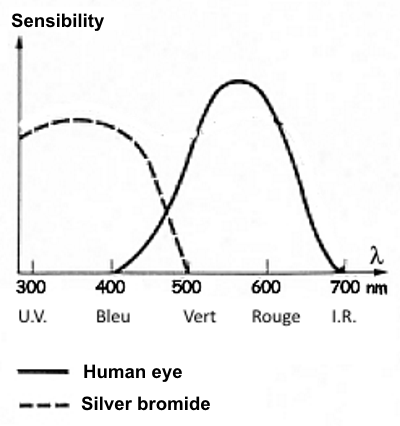### Photosensitizing

The sensitizers also absorb light and can transmit this energy gain to the excited electron and raise it in a higher quantum level ("conduction band") where it can move freely in the crystal and reach the silver ions to reduce them.$Ag^+$ $+$ $e^-$ $\longrightarrow$ $Ag$

### Germs

Few silver atoms formed in that manner flow in the crystal gaps and tend to clump on the surface by gaining lattice energy (energy from the formation of the metallic bond). It was found that these germs preferentially form where impurities such as sulfur are condensation nuclei. The germs are extremely small. You can not see them with the naked eye. They are said to form the latent image.## Development

Once exposed, the film is processed in the darkroom:

### Revelation

The revelation is the process intended to form around the germs a large amount of silver atoms to achieve a real image:For this purpose, the film is introduced into a bath consisting of - hydroquinone (developer) - sodium carbonate - potassium bromide (a bit) Hydroquinone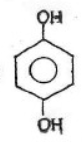is a reductant The redox systems are: (1)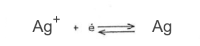(2)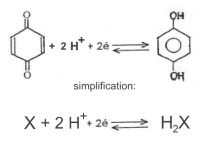with following standard redox potentials: $E^o_1=0,80\; V$ $E^o_2=0,69\; V$ Here we are far from standard conditions, so that we must consider →       Nernst's law : (a) $E_1$ $=$ $E^o_1$ $+$ $\frac{0,059}{1}log[Ag^+]$ (b) $E_2$ $=$ $E^o_2$ $+$ $\frac{0,059}{2}log\frac{[X][H^+]^2}{[H_2X]}$ Due to the very low solubility of silver bromide, $log[Ag^+]$ becomes negative and the real potential $E_1$ of system (a) is significantly decreased. The added basic carbonate ions decreases $[H^+]$: $CO_3^{2-}$ $+$ $H^+$ $\longrightarrow$ $HCO_3^-$ and thus lowers the potential $E_2$ of system (b) below $E_1$! So hydroquinone can reduce silver ions into silver atoms: $2Ag^+$ $+$ $2e^-$ $\longrightarrow$ $2Ag$ $C_6H_4(OH)_2$ $+$ $2OH^-$ $-$ $2e^-$ $\longrightarrow$ $C_6H_4O_2$ $+$ $2H_2O$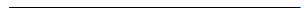$2Ag^+$ $+$ $C_6H_4(OH)_2$ $+$ $2OH^-$ $\longrightarrow$ $C_6H_4O_2$ $+$ $2H_2O$ Silver Ion + Hydroquinone $\longrightarrow$ quinone and silver atom

### The veil

The free $Ag^+$ ions remaining in the emulsion can cause a gray veil ($Ag$) when exposed to light. Potassium bromide decreases their molarity and prevents the veil. Indeed: $[Ag^+][Br^-]=K_s$ where $K_S$ is the solubility product of silver bromide. If $[Br -]$ increases as a result of the addition of potassium bromide, $[Ag ^ +]$ must decrease.

### The stop bath

If hydroquinone is present too long a time, the photograph may become dark. Its action is stopped after a given time by acidifying the emulsion and thus raising its redox potential (see above). For this, the film is introduced into a bath of dilute acetic acid.

### The fixing

Silver bromide that has not been turned into silver may darken when the picture is out of the darkroom. It is eliminated by complexing with the thiosulfate ion $S_2O_3^{2 -}$. For this, the film is introduced into a fixing bath of dilute sodium thiosulfate.

$Ag^+Br^-$ $+$ $4Na^+$ $+$ $2S_2O_3^{2-}$ $\longrightarrow$ $3Na^+$ $+$ $[Ag(S_2O_3)_2]^{3-}$ $+$ $3Na^+$ $+$ $Br^-$

Sodium bromide and sodium silverdithiosulfate are soluble in water and can therefore be removed by washing.

### The washing

Development ends by intense washing with distilled water followed by drying.→   Summary